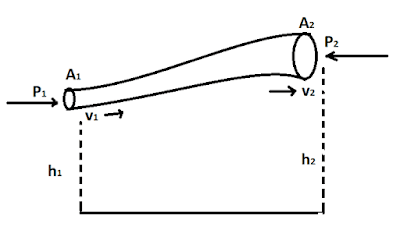10 Assumption of Bernoulli's Theorem - Civil Engineering

10 Assumption of Bernoulli's Theorem

Following are the 10 assumptions of Bernoulli's theorem:10 Assumption of Bernoulli's Theorem
1. Velocity is uniform.
2. Flow is uniform.
3. Flow is steady.
4. Flow is irrotational.
5. The fluid is incompressible.
6. Flow is caused only by gravity force.
7. There s no loss of energy in the flow.
8. Centrifugal force in the curve path is neglected.
9. Frictional, as well as viscous drag forces, are also neglected.
10. Liquid flow in a continuous stream.